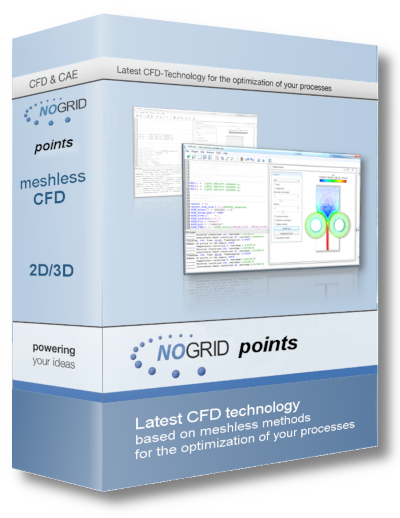# CFD Lexicon

## Glossary of Terms for NOGRID's Computational Fluid Dynamics (CFD)

Software solutions for authoring product design.

CAE Acronym for Computer-Aided Engineering
Software solutions for simulating the physical behavior of a future product.

CAM Acronym for Computer-Aided Manufacturing
Software solutions for defining manufacturing operations.

CFD Acronym for Computational Fluid Dynamics
CFD is a computer-based mathematical modelling tool that incorporates the solution of the fundamental equations of fluid flow, the Navier-Stokes equations and other allied equations. CFD incorporates empirical models for modelling turbulence based on experimentation, as well as the solution of heat, mass and other transport and field equations. There are usually four steps required to compute and anylyse a CFD case:
1) preprocessing, or creation of a geometry usually done in a CAD tool
2) mesh generation of a suitable computational domain to solve the flow equations on
3) solving with postprocessing
4) visualization of the CFD results
The main advantage of NOGRID CFD code is to avoid the second step: mesh generation. NOGRID codes use a point cloud instead which is more flexible and well prepared for today's and future modelling tasks. CFD is now a widely accepted and validated engineering tool for industrial applications.

DEM Acronym for Discrete Element Modeling
This is a numerical technique which solves engineering problems that are modeled as a large system of distinct interacting general shaped (deformable or rigid) bodies or particles that are subject to gross motion. Engineering problems that exhibit such large scale discontinuous behavior cannot be solved with a conventional continuum based procedure such as the Finite Element Method.

DNS Acronym for Direct Numerical Simulation
This is a CFD method by which the complete turbulent flow field is solved directly numerically without any form of time or length averaging in the domain, i.e. both the mean flow and all turbulent eddies (fluctuations) are simulated. DNS is very computationally intensive and currently only practicable for simple flows at low Reynolds Numbers.

FEM Acronym for Finite Element Method
This is a numerical technique for solving boundary-value problems. In a fluid flow application, the flow domain can be represented by finite elements, i.e. a geometrically similar model consists of several simplified discrete regions, which are linked. Flow governing equations (mass and momentum conservation, constitutive equations) are applied to each element and a system of equations is constructed in terms of nodal unknowns. Techniques of linear algebra, combined with non-linear numerical schemes are subsequently invoked for solving the system.

FPM Acronym for Finite Pointset Method
This is a numerical technique for solving partial differential equations (PDE) that uses a point cloud in oder to solve the equations. It based on a general finite difference scheme.

FVM Acronym for Finite Volume Method
This is a method for representing and evaluating partial differential equations (PDE) as algebraic equations.

FSI Acronym for Fluid-Structure Interaction
This is a model that allows for the deformation of solid boundaries to be taken into account when there is physical interaction with a flow field. This is relevant in applications where deformations undergone by boundaries are significant and affect the flow domain. FSI encompasses those physical phenomena for which aerodynamic (hydrodynamic), elastic (structural), and inertial (dynamic) forces influence each other and interact in a significant way. Aeroelasticity (Hydroelasticity) is an important subset of fluid-structure interaction. Flutter in aircraft is a subset of aeroelasticity. Vortex-Induced Vibration (VIV) is a subset of hydroelasticity. Modelling FSI can usually be achieved by coupling a CFD code with a structural analysis code.

GUI Acronym for Graphical User Interface
This is a computer screen interface that presents information (often applications, i.e. CFD codes) in a user-friendly way using pictures, graphics and icons.

IGES Acronym for Initial Graphics Exchange Specification
This is an agreed international neutral file format used to translate geometrical information between different CAD, CAE and analysis software packages. Visit www.nist.gov/iges/ for details.

MECHANICAL DESIGN  The conception, modelization and design of mechanical systems. From concept to detailed design and on to drawing production, mechanical design products accelerate core activities of product development. Mechanical design products also address sheet metal and mold manufacturer requirements through dedicated applications that dramatically enhance productivity and strongly reduce time-to-market.

POINT CLOUD In FPM terminology the point distribution within the fluid domain. A point cloud is a set of mathematical particles in a two or three dimensional space. FPM points are used to descretize the govern differential equations. Discretization concerns the process of transferring continuous models and equations into discrete counterparts. This process is usually carried out as a first step toward making them suitable for numerical calculations.

STEP Acronym for Standard for the Exchange of Product Model Data
This is an international standard for the exchange of digital product information (ISO 10303). Visit www.steptools.com/library/standard/ for details.

VOF Acronym for Volume of Fluid/Flow Model
The VOF model is used mainly for multiphase flows, and in particular free surface (or wave) flows.

Göttelmannstr. 13B
55130 Mainz, Germany
info@nogrid.comNogrid